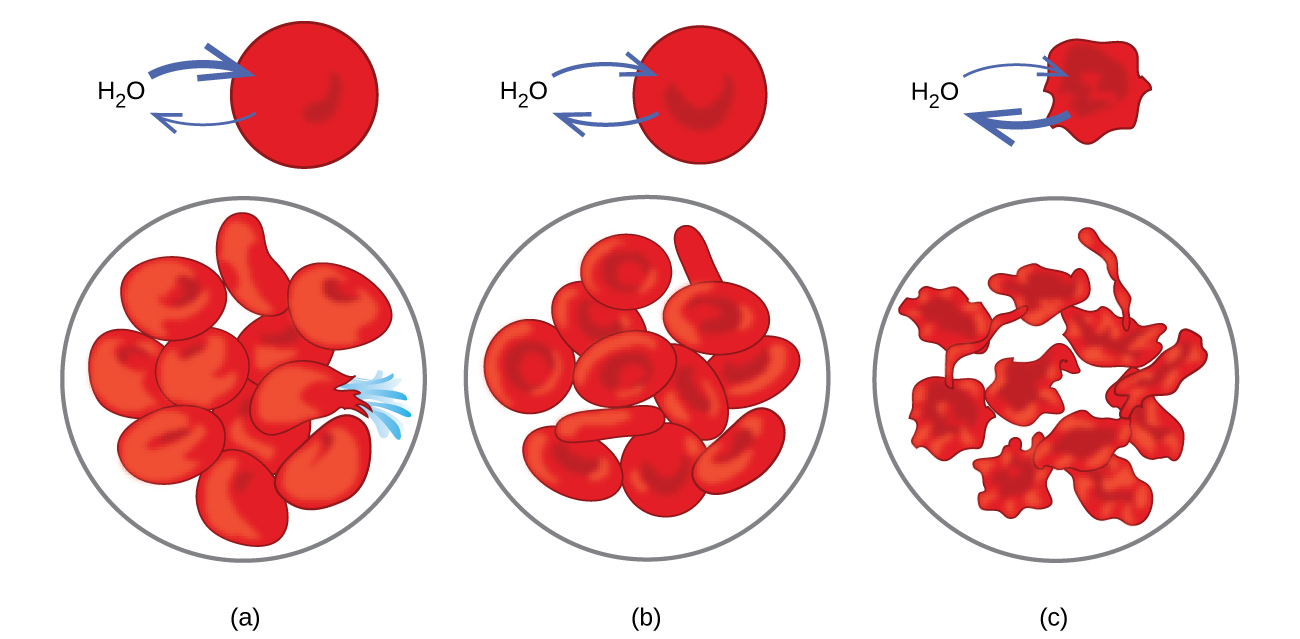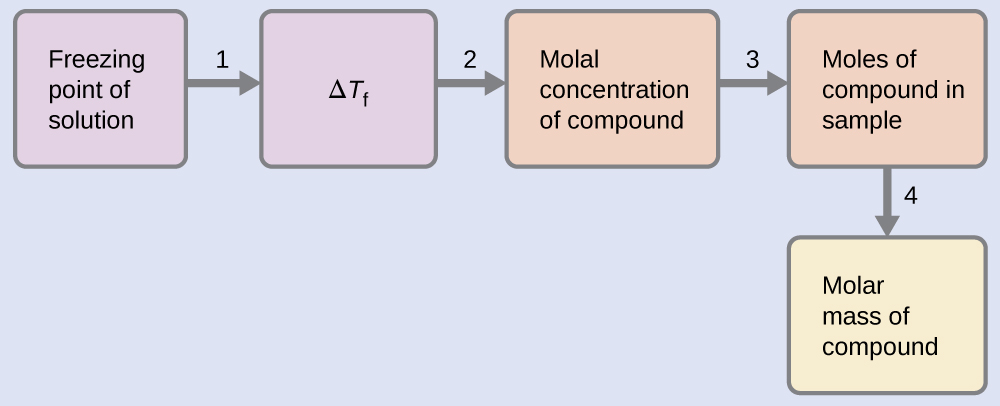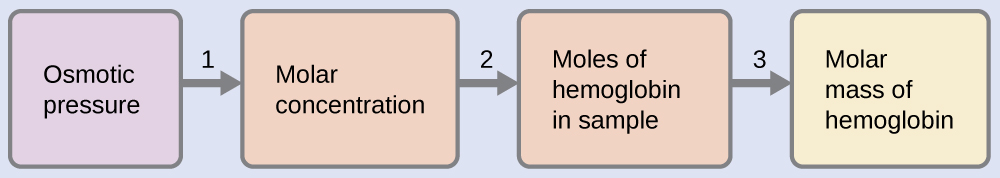# 6.4 Colligative properties  (Page 8/30)

 Page 8 / 30Red blood cell membranes are water permeable and will (a) swell and possibly rupture in a hypotonic solution; (b) maintain normal volume and shape in an isotonic solution; and (c) shrivel and possibly die in a hypertonic solution. (credit a/b/c: modifications of work by “LadyofHats”/Wikimedia commons)

## Determination of molar masses

Osmotic pressure and changes in freezing point, boiling point, and vapor pressure are directly proportional to the concentration of solute present. Consequently, we can use a measurement of one of these properties to determine the molar mass of the solute from the measurements.

## Determination of a molar mass from a freezing point depression

A solution of 4.00 g of a nonelectrolyte dissolved in 55.0 g of benzene is found to freeze at 2.32 °C. What is the molar mass of this compound?

## Solution

We can solve this problem using the following steps.1. Determine the change in freezing point from the observed freezing point and the freezing point of pure benzene ( [link] ).
$\text{Δ}{T}_{\text{f}}=5.5\phantom{\rule{0.2em}{0ex}}\text{°}\text{C}-2.32\phantom{\rule{0.2em}{0ex}}\text{°}\text{C}=3.2\phantom{\rule{0.2em}{0ex}}\text{°}\text{C}$
2. Determine the molal concentration from K f , the freezing point depression constant for benzene ( [link] ), and Δ T f .
$\begin{array}{l}\hfill \text{Δ}{T}_{\text{f}}={K}_{\text{f}}m\hfill \\ \\ m\phantom{\rule{0.2em}{0ex}}=\phantom{\rule{0.2em}{0ex}}\frac{\text{Δ}{T}_{\text{f}}}{{K}_{\text{f}}}\phantom{\rule{0.2em}{0ex}}=\phantom{\rule{0.2em}{0ex}}\frac{3.2\phantom{\rule{0.2em}{0ex}}\text{°}\text{C}}{5.12\phantom{\rule{0.2em}{0ex}}\text{°}\text{C}\phantom{\rule{0.2em}{0ex}}{m}^{-1}}\phantom{\rule{0.2em}{0ex}}=\phantom{\rule{0.2em}{0ex}}0.63\phantom{\rule{0.2em}{0ex}}m\end{array}$
3. Determine the number of moles of compound in the solution from the molal concentration and the mass of solvent used to make the solution.
$\text{Moles of solute}\phantom{\rule{0.2em}{0ex}}=\phantom{\rule{0.2em}{0ex}}\frac{0.62\phantom{\rule{0.2em}{0ex}}\text{mol solute}}{1.00\phantom{\rule{0.2em}{0ex}}\overline{)\text{kg solvent}}}\phantom{\rule{0.2em}{0ex}}×\phantom{\rule{0.2em}{0ex}}0.0550\phantom{\rule{0.2em}{0ex}}\overline{)\text{kg solvent}}=0.035\phantom{\rule{0.2em}{0ex}}\text{mol}$
4. Determine the molar mass from the mass of the solute and the number of moles in that mass.
$\text{Molar mass}\phantom{\rule{0.2em}{0ex}}=\phantom{\rule{0.2em}{0ex}}\frac{4.00\phantom{\rule{0.2em}{0ex}}\text{g}}{0.034\phantom{\rule{0.2em}{0ex}}\text{mol}}\phantom{\rule{0.2em}{0ex}}=\phantom{\rule{0.2em}{0ex}}1.2\phantom{\rule{0.2em}{0ex}}×\phantom{\rule{0.2em}{0ex}}{10}^{2}\text{g/mol}$

A solution of 35.7 g of a nonelectrolyte in 220.0 g of chloroform has a boiling point of 64.5 °C. What is the molar mass of this compound?

1.8 $×$ 10 2 g/mol

## Determination of a molar mass from osmotic pressure

A 0.500 L sample of an aqueous solution containing 10.0 g of hemoglobin has an osmotic pressure of 5.9 torr at 22 °C. What is the molar mass of hemoglobin?

## Solution

Here is one set of steps that can be used to solve the problem:1. Convert the osmotic pressure to atmospheres, then determine the molar concentration from the osmotic pressure.
$\begin{array}{}\\ \Pi \phantom{\rule{0.2em}{0ex}}=\phantom{\rule{0.2em}{0ex}}\frac{5.9\phantom{\rule{0.2em}{0ex}}\text{torr}\phantom{\rule{0.2em}{0ex}}×\phantom{\rule{0.2em}{0ex}}1\phantom{\rule{0.2em}{0ex}}\text{atm}}{760\phantom{\rule{0.2em}{0ex}}\text{torr}}\phantom{\rule{0.2em}{0ex}}=\phantom{\rule{0.2em}{0ex}}7.8\phantom{\rule{0.2em}{0ex}}×\phantom{\rule{0.2em}{0ex}}{10}^{-3}\text{atm}\\ \Pi =\mathit{\text{MRT}}\\ \\ M\phantom{\rule{0.2em}{0ex}}=\phantom{\rule{0.2em}{0ex}}\frac{\Pi }{RT}\phantom{\rule{0.2em}{0ex}}=\phantom{\rule{0.2em}{0ex}}\frac{7.8\phantom{\rule{0.2em}{0ex}}×\phantom{\rule{0.2em}{0ex}}{10}^{-3}\phantom{\rule{0.2em}{0ex}}\text{atm}}{\left(0.08206\phantom{\rule{0.2em}{0ex}}\text{L atm/mol K}\right)\left(295\phantom{\rule{0.2em}{0ex}}\text{K}\right)}\phantom{\rule{0.2em}{0ex}}=\phantom{\rule{0.2em}{0ex}}3.2\phantom{\rule{0.2em}{0ex}}×\phantom{\rule{0.2em}{0ex}}{10}^{-4}\text{M}\end{array}$
2. Determine the number of moles of hemoglobin in the solution from the concentration and the volume of the solution.
$\text{moles of hemoglobin}\phantom{\rule{0.2em}{0ex}}=\phantom{\rule{0.2em}{0ex}}\frac{3.2\phantom{\rule{0.2em}{0ex}}×\phantom{\rule{0.2em}{0ex}}{10}^{-4}\text{mol}}{1\phantom{\rule{0.2em}{0ex}}\overline{)\text{L solution}}}\phantom{\rule{0.2em}{0ex}}×\phantom{\rule{0.2em}{0ex}}0.500\phantom{\rule{0.2em}{0ex}}\overline{)\text{L solution}}=1.6\phantom{\rule{0.2em}{0ex}}×\phantom{\rule{0.2em}{0ex}}{10}^{-4}\text{mol}$
3. Determine the molar mass from the mass of hemoglobin and the number of moles in that mass.
$\text{molar mass}\phantom{\rule{0.2em}{0ex}}=\phantom{\rule{0.2em}{0ex}}\frac{10.0\phantom{\rule{0.2em}{0ex}}\text{g}}{1.6\phantom{\rule{0.2em}{0ex}}×\phantom{\rule{0.2em}{0ex}}{10}^{-4}\text{mol}}\phantom{\rule{0.2em}{0ex}}=\phantom{\rule{0.2em}{0ex}}6.2\phantom{\rule{0.2em}{0ex}}×\phantom{\rule{0.2em}{0ex}}{10}^{4}\text{g/mol}$

What is the molar mass of a protein if a solution of 0.02 g of the protein in 25.0 mL of solution has an osmotic pressure of 0.56 torr at 25 °C?

2.7 $×$ 10 4 g/mol

## Colligative properties of electrolytes

As noted previously in this module, the colligative properties of a solution depend only on the number, not on the kind, of solute species dissolved. For example, 1 mole of any nonelectrolyte dissolved in 1 kilogram of solvent produces the same lowering of the freezing point as does 1 mole of any other nonelectrolyte. However, 1 mole of sodium chloride (an electrolyte) forms 2 moles of ions when dissolved in solution. Each individual ion produces the same effect on the freezing point as a single molecule does.

where we get a research paper on Nano chemistry....?
what are the products of Nano chemistry?
There are lots of products of nano chemistry... Like nano coatings.....carbon fiber.. And lots of others..
learn
Even nanotechnology is pretty much all about chemistry... Its the chemistry on quantum or atomic level
learn
da
no nanotechnology is also a part of physics and maths it requires angle formulas and some pressure regarding concepts
Bhagvanji
hey
Giriraj
Preparation and Applications of Nanomaterial for Drug Delivery
revolt
da
Application of nanotechnology in medicine
what is variations in raman spectra for nanomaterials
I only see partial conversation and what's the question here!
what about nanotechnology for water purification
please someone correct me if I'm wrong but I think one can use nanoparticles, specially silver nanoparticles for water treatment.
Damian
yes that's correct
Professor
I think
Professor
Nasa has use it in the 60's, copper as water purification in the moon travel.
Alexandre
nanocopper obvius
Alexandre
what is the stm
is there industrial application of fullrenes. What is the method to prepare fullrene on large scale.?
Rafiq
industrial application...? mmm I think on the medical side as drug carrier, but you should go deeper on your research, I may be wrong
Damian
How we are making nano material?
what is a peer
What is meant by 'nano scale'?
What is STMs full form?
LITNING
scanning tunneling microscope
Sahil
how nano science is used for hydrophobicity
Santosh
Do u think that Graphene and Fullrene fiber can be used to make Air Plane body structure the lightest and strongest. Rafiq
Rafiq
what is differents between GO and RGO?
Mahi
what is simplest way to understand the applications of nano robots used to detect the cancer affected cell of human body.? How this robot is carried to required site of body cell.? what will be the carrier material and how can be detected that correct delivery of drug is done Rafiq
Rafiq
if virus is killing to make ARTIFICIAL DNA OF GRAPHENE FOR KILLED THE VIRUS .THIS IS OUR ASSUMPTION
Anam
analytical skills graphene is prepared to kill any type viruses .
Anam
Any one who tell me about Preparation and application of Nanomaterial for drug Delivery
Hafiz
what is Nano technology ?
write examples of Nano molecule?
Bob
The nanotechnology is as new science, to scale nanometric
brayan
nanotechnology is the study, desing, synthesis, manipulation and application of materials and functional systems through control of matter at nanoscale
Damian
Is there any normative that regulates the use of silver nanoparticles?
what king of growth are you checking .?
Renato
What fields keep nano created devices from performing or assimulating ? Magnetic fields ? Are do they assimilate ?
why we need to study biomolecules, molecular biology in nanotechnology?
?
Kyle
yes I'm doing my masters in nanotechnology, we are being studying all these domains as well..
why?
what school?
Kyle
biomolecules are e building blocks of every organics and inorganic materials.
Joe
how do you find theWhat are the wavelengths and energies per photon of two lines
The eyes of some reptiles are sensitive to 850 nm light. If the minimum energy to trigger the receptor at this wavelength is 3.15 x 10-14 J, what is the minimum number of 850 nm photons that must hit the receptor in order for it to be triggered?
A teaspoon of the carbohydrate sucrose contains 16 calories, what is the mass of one teaspoo of sucrose if the average number of calories for carbohydrate is 4.1 calories/g?
4. On the basis of dipole moments and/or hydrogen bonding, explain in a qualitative way the differences in the boiling points of acetone (56.2 °C) and 1-propanol (97.4 °C), which have similar molar masses
Calculate the bond order for an ion with this configuration: (?2s)2(??2s)2(?2px)2(?2py,?2pz)4(??2py,??2pz)3
Which of the following will increase the percent of HF that is converted to the fluoride ion in water? (a) addition of NaOH (b) addition of HCl (c) addition of NaFBy OpenStaxBy OpenStaxBy Sarah WarrenBy Ellie BanfieldBy Abby SharpBy OpenStaxBy OpenStaxBy Anonymous UserBy Yacoub JayoghliBy Angela Eckman